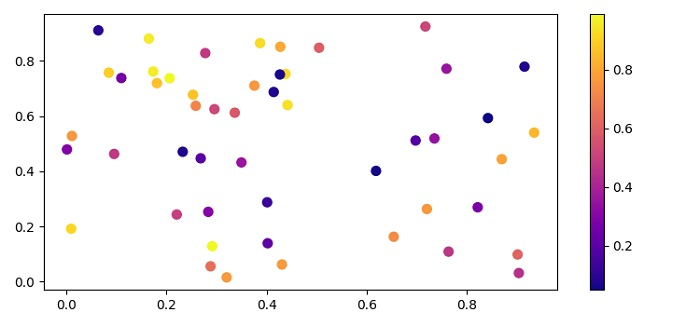# How to color a Matplotlib scatterplot using a continuous value?

To color a matplotlib scatterplot using continuous value, we can take the following steps −

• Set the figure size and adjust the padding between and around the subplots.

• Create x, y and z random data points using numpy.

• Create a figure and a set of subplots.

• Create a scatter plot.

• Draw a colorbar in an existing axes, with scatter points scalar mappable instance.

• To display the figure, use show() method.

## Example

import numpy as np
import matplotlib.pyplot as plt

plt.rcParams["figure.figsize"] = [7.50, 3.50]
plt.rcParams["figure.autolayout"] = True

x, y, z = np.random.rand(3, 50)
f, ax = plt.subplots()

points = ax.scatter(x, y, c=z, s=50, cmap="plasma")
f.colorbar(points)

plt.show()

## Output